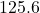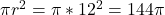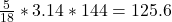## A sprinkler sprays in a circular pattern at a radius of 12 meters. If the degree of the circle that is covered is 100°, what is the ar

Question

A sprinkler sprays in a circular pattern at a radius of 12 meters. If the degree of the circle that
is covered is 100°, what is the area of the lawn that is covered by the water from the
sprinker? (Round to the nearest hundredth of a meter)

in progress 0
5 months 2021-08-23T10:34:31+00:00 1 Answers 2 views 0

The area of the lawn that is covered by the water from the  sprinkler issquare meter

Step-by-step explanation:

Given

The radius of the circle is 12 m

Area of the circle isFor one complete circle,  the degree of rotation is 360 degrees.

Area of lawn covered by rotation of 100 degrees only is 100/360 = 5/18

The area of the lawn that is covered by the water from the  sprinkler issquare meter